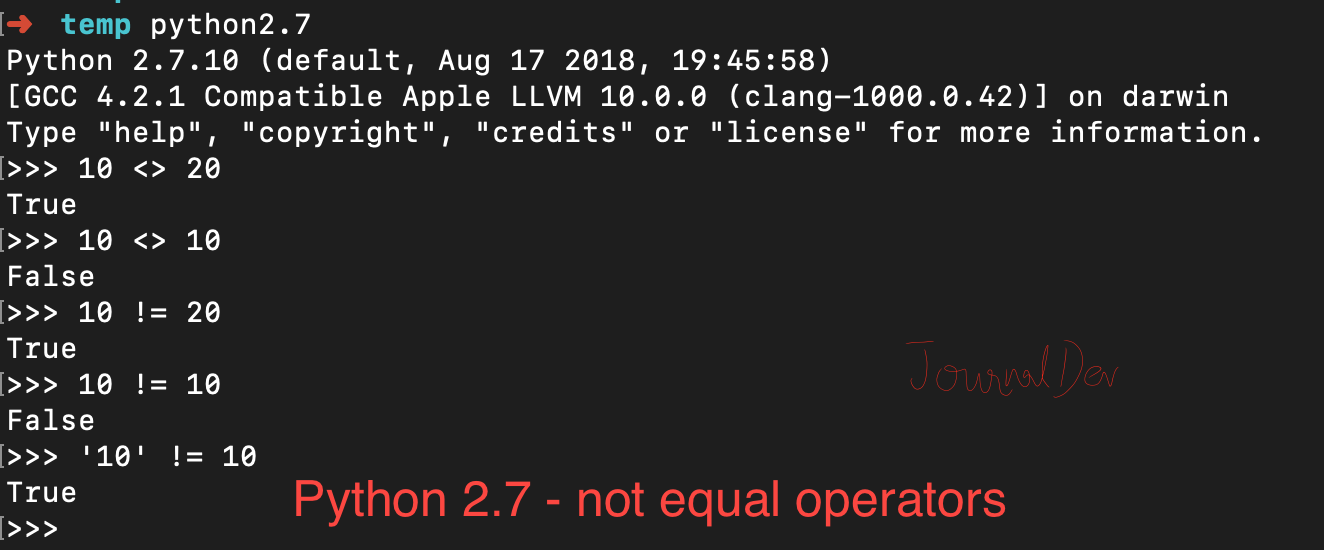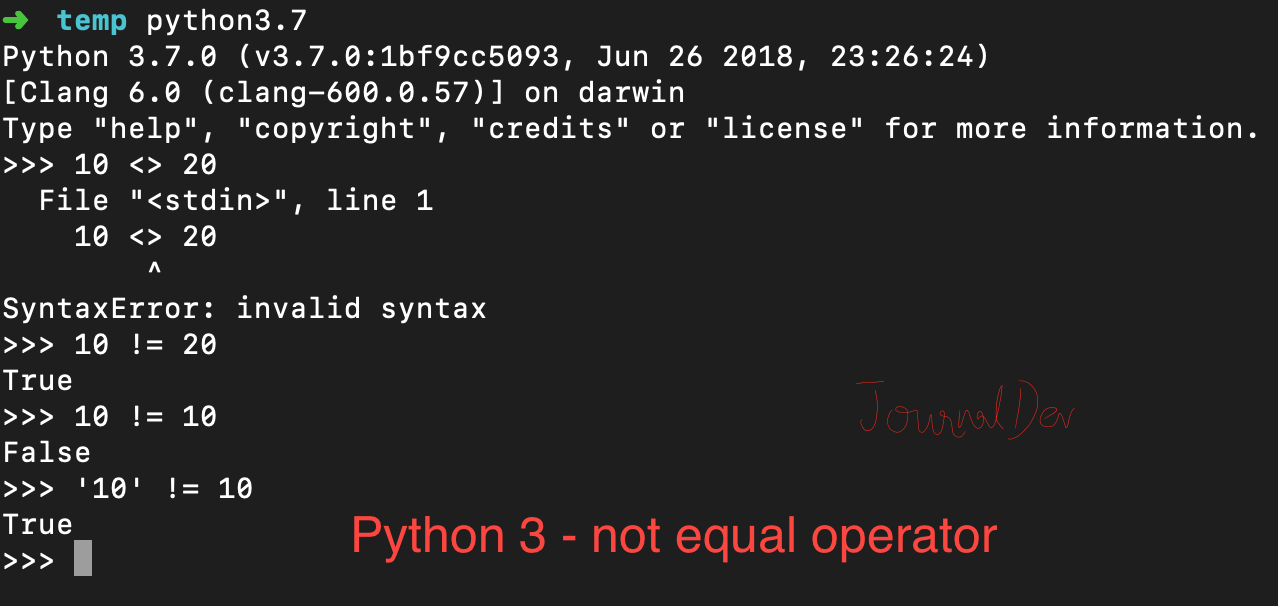# Python not equal operator

Published on August 3, 2022By Pankaj
Developer and author at DigitalOcean.While we believe that this content benefits our community, we have not yet thoroughly reviewed it. If you have any suggestions for improvements, please let us know by clicking the “report an issue“ button at the bottom of the tutorial.

Python not equal operator returns `True` if two variables are of same type and have different values, if the values are same then it returns `False`. Python is dynamic and strongly typed language, so if the two variables have the same values but they are of different type, then not equal operator will return `True`.

## Python not equal operators

Operator Description
!= Not Equal operator, works in both Python 2 and Python 3.
<> Not equal operator in Python 2, deprecated in Python 3.

## Python 2 Example

Let’s see some examples of not-equal operator in Python 2.7.

``````\$ python2.7
Python 2.7.10 (default, Aug 17 2018, 19:45:58)
[GCC 4.2.1 Compatible Apple LLVM 10.0.0 (clang-1000.0.42)] on darwin
>>> 10 <> 20
True
>>> 10 <> 10
False
>>> 10 != 20
True
>>> 10 != 10
False
>>> '10' != 10
True
>>>
``````## Python 3 Example

Here is some examples with Python 3 console.

``````\$ python3.7
Python 3.7.0 (v3.7.0:1bf9cc5093, Jun 26 2018, 23:26:24)
[Clang 6.0 (clang-600.0.57)] on darwin
>>> 10 <> 20
File "<stdin>", line 1
10 <> 20
^
SyntaxError: invalid syntax
>>> 10 != 20
True
>>> 10 != 10
False
>>> '10' != 10
True
>>>
``````We can use Python not equal operator with f-strings too if you are using Python 3.6 or higher version.

``````x = 10
y = 10
z = 20

print(f'x is not equal to y = {x!=y}')

flag = x != z
print(f'x is not equal to z = {flag}')

# python is strongly typed language
s = '10'
print(f'x is not equal to s = {x!=s}')
``````

Output:

``````x is not equal to y = False
x is not equal to z = True
x is not equal to s = True
``````

## Python not equal with custom object

When we use not equal operator, it calls `__ne__(self, other)` function. So we can define our custom implementation for an object and alter the natural output. Let’s say we have `Data` class with fields - id and record. When we are using the not-equal operator, we just want to compare it for record value. We can achieve this by implementing our own __ne__() function.

``````class Data:
id = 0
record = ''

def __init__(self, i, s):
self.id = i
self.record = s

def __ne__(self, other):
# return true if different types
if type(other) != type(self):
return True
if self.record != other.record:
return True
else:
return False

d1 = Data(1, 'Java')
d2 = Data(2, 'Java')
d3 = Data(3, 'Python')

print(d1 != d2)
print(d2 != d3)
``````

Output:

``````False
True
``````

Notice that d1 and d2 record values are same but “id” is different. If we remove __ne__() function, then the output will be like this:

``````True
True
``````

You can checkout complete python script and more Python examples from our GitHub Repository.

Thanks for learning with the DigitalOcean Community. Check out our offerings for compute, storage, networking, and managed databases.Pankaj

author

Developer and author at DigitalOcean.

#### Still looking for an answer?

Ask a questionSearch for more help

JournalDev
DigitalOcean EmployeeMarch 11, 2020

Great explanation Pankaj! Thanks

- Nico